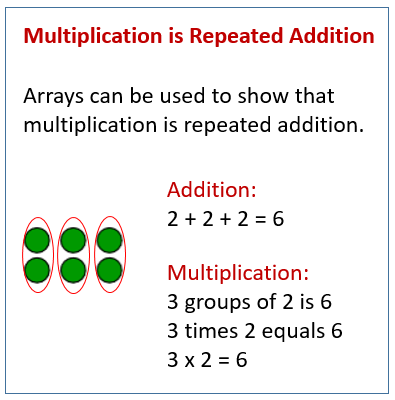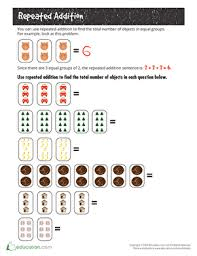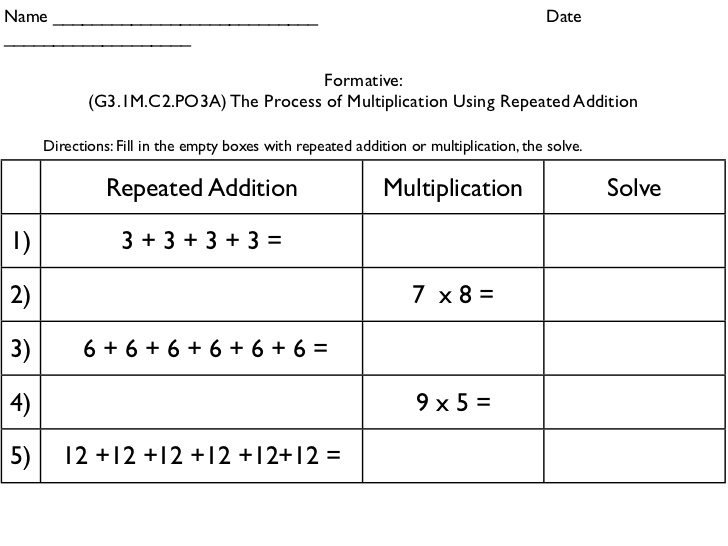# MULTIPLICATION BY USING REPEATED ADDITION

HELLO CHILDREN, WELCOME BACK TO CLASS

Repeated addition is also known as multiplication. If the same number is repeated then in short, we can write that in the form of multiplication.

For example: 2 + 2 + 2 + 2 + 2

Here 2 is repeated 5 times so in short, we can write this addition as 2 x 5.If there are 8 friends and 2 balloons are given to each friend then 2 + 2 + 2 + 2 + 2 + 2 + 2 + 2 = 16 This repeated-addition can be written in the form of multiplication as 8 x 2 = 16

So, multiplication is easier than repeated-addition.ACTIVITYYour Opinion Matters! Quickly tell us how to improve your Learning Experience HERE